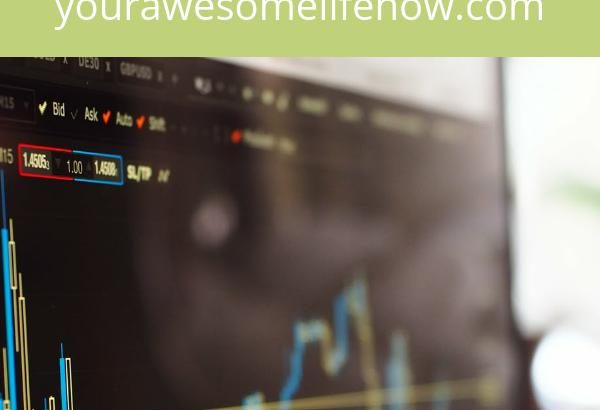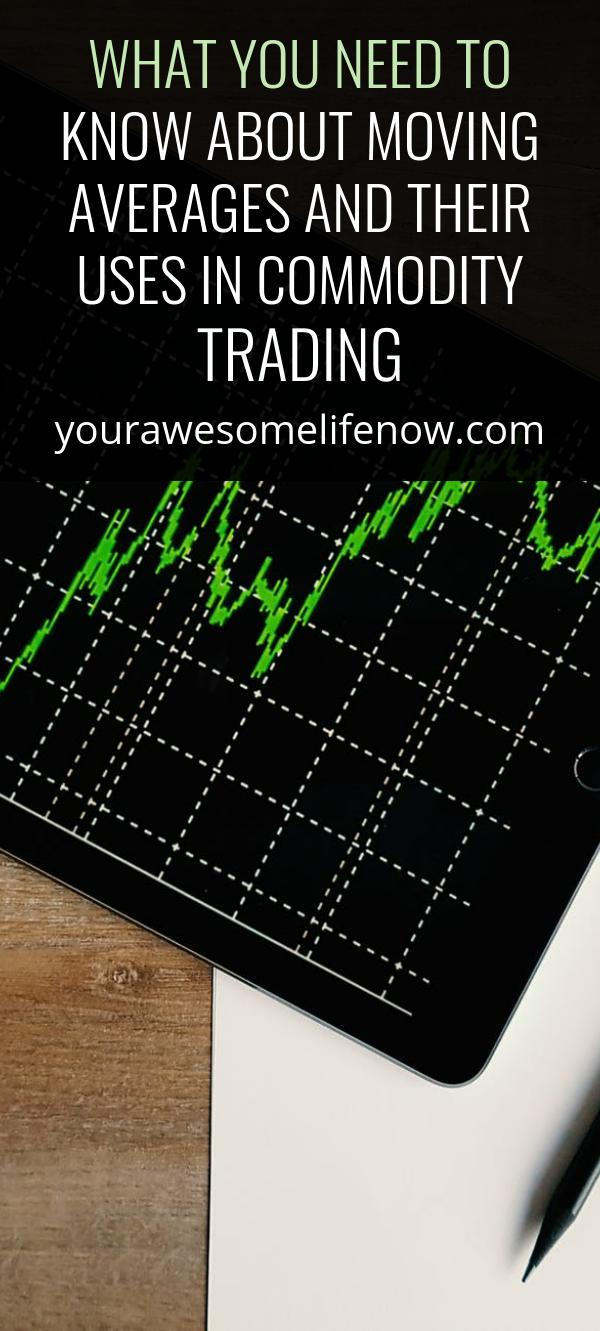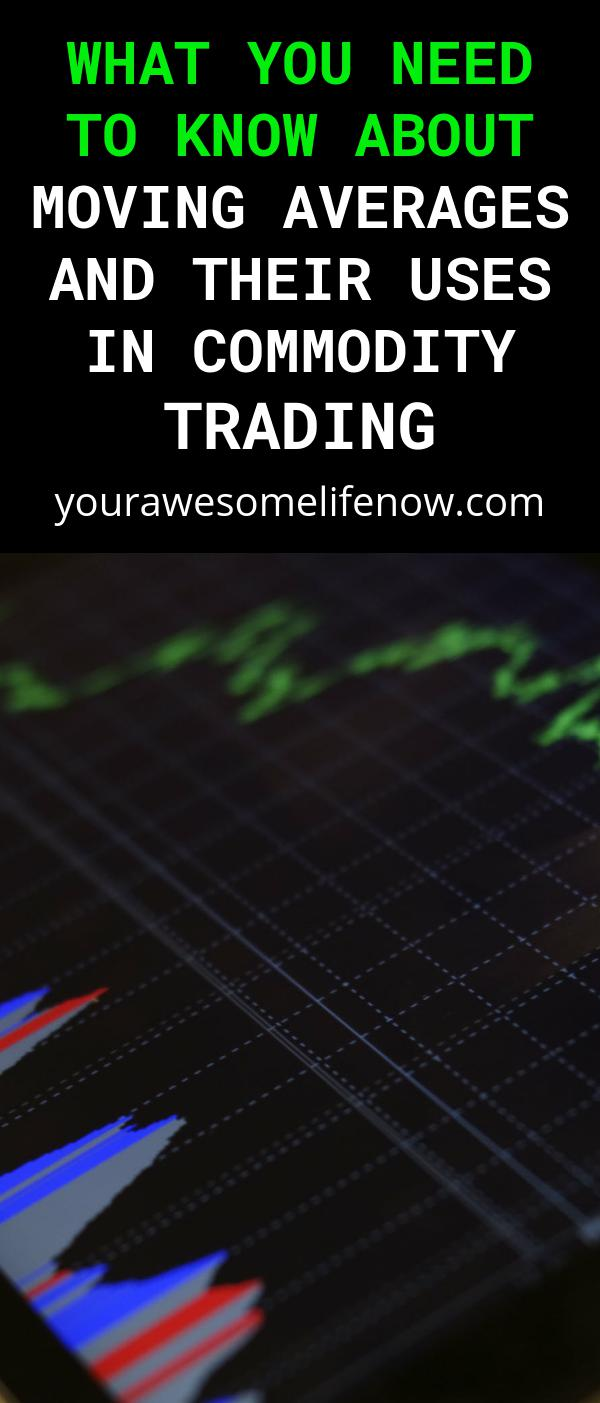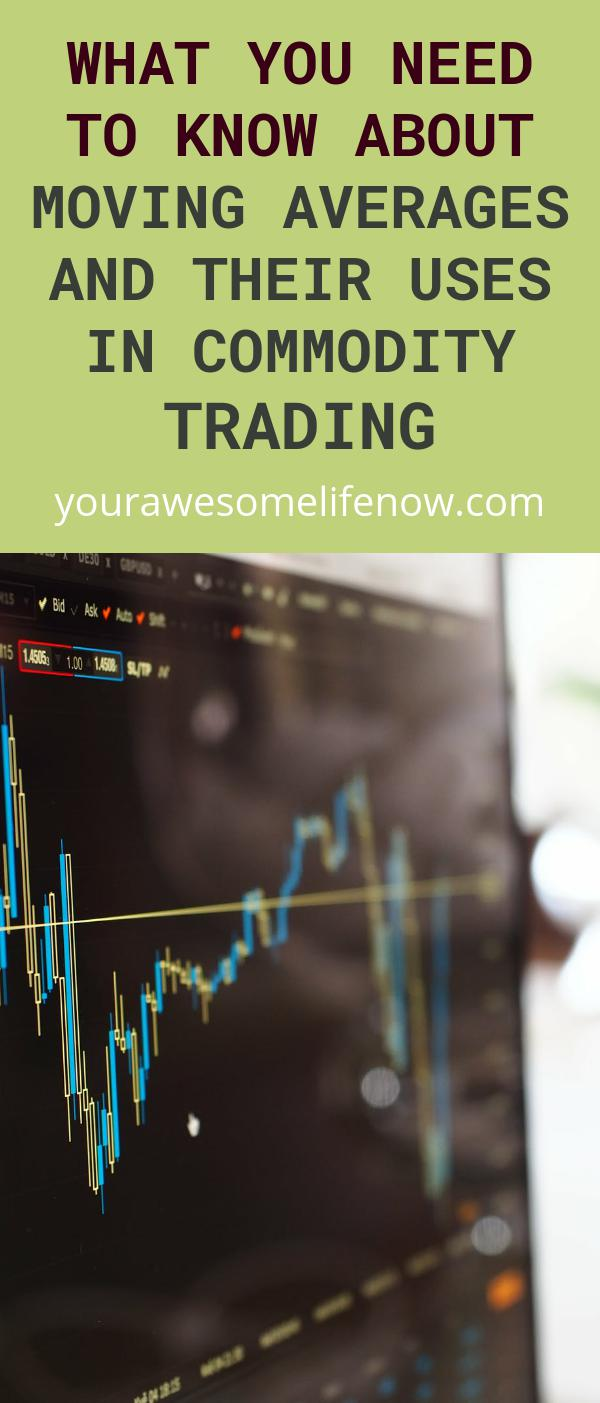A key component of technical analysis and perhaps one of the oldest indicators around, moving averages are time-tested and affective indicators. There are many types of moving averages with varying indicators, but the primary purpose of all types of moving averages remains the same. Their purpose is to reduce or remove noise from the daily price movements and attracted trends of stocks, commodities or anything you can plot or chart.

Moving Averages: How Do We Use them?Moving averages identify trends and trend reversals, give a measure of a commodities strength, and help you arrive at support and resistance levels. Essentially, moving averages are indicators with lag, which is to say that they do not identify new trends but are useful in trend following. One of the most useful ways in which you can use moving averages as buy or sell indicators, is to have three moving averages running at the same time on the same chart. The idea is to have a short, an intermediate and a longer term time frame. When the first two move upwards and cross above the longer term one, it indicates an uptrend and one can buy. The reverse happens if the first two move below the third moving average. In that case, you can sell, as the commodity is in a downtrend. A good example of this would be a 10, 20, and a 30 day period moving average, plotted on a commodity chart.

Moving averages are also used by traders to determine support and resistance of a commodity. When the commodity reaches a moving average and struggles to move above it, you might have found resistance. If a commodity stops falling at a key moving average, it can be deemed to have found support. A prime example of this is a 200 day moving average, which is used to calculate long-term trend directions, and to find support and resistance in them.

Types of Moving AveragesThere are different types of moving averages. The simplest one is the simple moving average (SMA), which is calculated by taking the normal arithmetic mean of a specified set of numbers. The exponential moving average (EMA) is calculated by giving weightage to more recent data. The EMA is regarded to be a better moving average compared to the SMA. Both of these moving average variants become very useful when used for trend following with moving average crossovers. Indicators such as the moving average convergence divergence (MACD) and Bollinger bands use moving averages as key components. The MACD shows the price divergence of two moving averages, by subtracting a 26 period EMA from the 12 period EMA. A third 9 period EMA is used to give us buy or sell signals when it moves above or below this MACD. Bollinger bands, so named after their creator, use two standard deviations plotted away from a 21 period SMA.

Whichever way you look at it, one cannot deny that using moving averages by themselves may not make you a millionaire in a hurry, but are brilliantly useful in helping you follow trends and plan your commodity trading strategy.